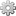# MathJax MacroEnter content supported by the MathJax javascript engine
Recommended
 Type XAR Category Macro Developed by Active Installs 22 Rating 1 Votes License GNU Lesser General Public License 2.1
 Compatibility Since v1.1 requires XWiki 14.9

Installable with the Extension Manager

# Description

A macro that allows entering content interpreted by the MathJax javascript library.

Note that MathJax allows entering equations but it also supports a lot more, such as equation references, and more.

# Usage

Example usage (input in xwiki/2.1 syntax):

Normal Wiki text followed by a formula

{{mathjax}}
\begin{eqnarray}
1 & = & a x + b y\label{eq:first} \\
0 & = & c x + d y\label{eq:second}
\end{eqnarray}
{{/mathjax}}

Solving equation {{mathjax}}\ref{eq:second}{{/mathjax}} for {{mathjax}}x{{/mathjax}} and substituting back into equation {{mathjax}}\ref{eq:first}{{/mathjax}} yields

{{mathjax}}
\begin{equation}
\end{equation}
{{/mathjax}}

Renders as:# LaTeX Export

This macro also provides a LaTeX template for the LaTeX extension, allowing the content to be rendered in LaTeX.

The example above is translated into the following LaTeX content when exported to LaTeX:

Normal Wiki text followed by a formula

\begin{eqnarray}
1 & = & a x + b y\label{eq:first} \\
0 & = & c x + d y\label{eq:second}
\end{eqnarray}

Solving equation \ref{eq:second} for x and substituting back into equation \ref{eq:first} yields

\begin{equation}
\end{equation}

# Prerequisites & Installation Instructions

We recommend using the Extension Manager to install this extension (Make sure that the text "Installable with the Extension Manager" is displayed at the top right location on this page to know if this extension can be installed with the Extension Manager). Note that installing Extensions when being offline is currently not supported and you'd need to use some complex manual method.

You can also use the following manual method, which is useful if this extension cannot be installed with the Extension Manager or if you're using an old version of XWiki that doesn't have the Extension Manager:

2. Go to the Administration page and select the Import category
5. You'll also need to install all dependent Extensions that are not already installed in your wiki

Bugfix

# Dependencies

Dependencies for this extension (org.xwiki.contrib.mathjax:macro-mathjax-ui 1.1.3):

Tags: